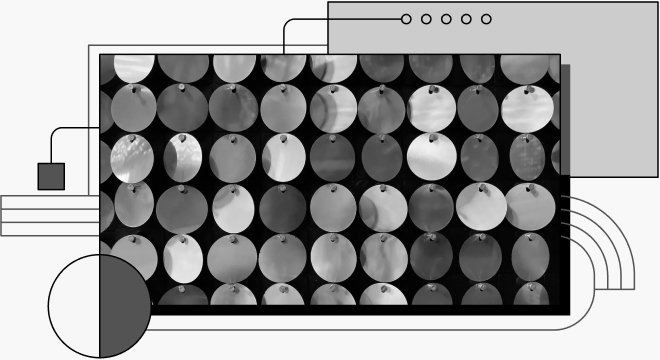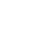# An Overview of Arrays as Data Structures in JavaAs a developer, you need to understand data structures and how they can help your tech journey. This article will solely focus on how arrays work as data structures. The primary programming language for this article will be Java.

## What are Data Structures?

Data structures are the formats for organizing, processing, retrieving, and storing data. They are designed to arrange data in a specific way that makes it easy for users to access the data they need.

Some benefits of data structures include, but are not limited to, the following:

• They help to secure data
• They help to organize data
• They allow for easy processing of data on a software system
• They help to solve real-life problems.

## Types of Data Structures

There are seven data structures every developer should know:

### Arrays

Arrays are famous data structures. An array is a collection of values that are stored chronologically. Values in an array have zero indexing, i.e., stored values start from 0.

A LinkedList is a data structure with nodes containing a data field that links to the next node in a list. The link is also called a reference.

### Stacks

Stacks are data structures that follow a specific order. The order could either be the First In, Last Out (FILO) or the Last In, First out (LIFO) order. They are also a linear data structure, i.e., the data structure is arranged in a line or follows a straight line trend.

### Queues

Queues are a linear data structure that opens at both ends and operations occur in a First In, First Out (FIFO) order.

### Trees

Similar to a family tree, trees are a collection of nodes. Each node of the tree stores a value and links to other nodes (children). This type of data structure, called a hierarchical structure, specializes in efficiently organizing and storing data.

### Heaps

Heaps are unique data structures— they’re entirely binary tree. There are two types of heaps:

1. Max heap property: This is where the heap is greater than the child node(s), and the key of the root node is more significant than other nodes.
2. Min heap property: This is where the heap is smaller than the child node(s), and the key of the root node is the smallest in the tree.

### Graphs

Graphs are data structures involving vertices (each shape's angular point) and edges. In a data structure, vertices are defined as nodes and edges as lines connecting two nodes. Ultimately, you can say that graphs make a set of vertices (V) and a bunch of edges (E); it’s denoted as G(E, V).

## Arrays as a Data Structure

As mentioned earlier, arrays are a collection of values stored in multiple values in a single variable instead of storing each value in a separate variable. To declare an array in Java, define the variable type followed by square brackets.

`String[] books:`

Save this code

An example of an array is a carton of eggs, because a carton has eggs lined up in a sequence and each section holds an egg.

Arrays are commonly used to build other, more complicated data structures. They are also used for sorting algorithms.

### Multi-dimensional arrays

These are simply arrays of arrays, which means one array inside another—multi-dimensional arrays store values/elements in a tabular form.

The syntax of multi-dimensional arrays:

`data_type[1st dimension][2nd dimension][]..[Nth dimension]array_name = new data_type[size1][size2]….[sizeN];`

Save this code

• `data_type` represents the data type, e.g., `int`, `String`, `char`
• `dimension` represents the dimension of arrays you want to create, e.g., 1D, 2D, etc.
• `array_name` represents the name of the array
• `size1`, `size2` represent the size of the dimensions

### Two-dimensional arrays

A two-dimensional array is an array of one-dimensional arrays. It is the simplest form of any multi-dimensional array.

An example of two multi-dimensional arrays:

`class example {    public static void main(String[] args)    {         int[][] arr = { { 5, 0 }, { 1, 7 } };        for (int i = 0; i < 2; i++) {            for (int j = 0; j < 2; j++) {                System.out.print(arr[i][j] + " ");            }             System.out.println();        }    }}`

Output:

`5 01 7`

### Three-dimensional arrays

A three-dimensional array is a complex form of multi-dimensional array because it has three sides/dimensions. An easy way to understand it is that this form of a multi-dimensional array is an array of two-dimensional arrays.

An example of three multi-dimensional arrays:

`class example {  public static void main(String[] args) {    int[][][] arr = {{{ 5, 0 }, { 1, 7 },{2 , 9}},};        for(int i=0; i < arr.length; i++){      for(int j=0; j < arr[i].length; j++){         for(int k=0; k < arr[i][j].length; k++){            System.out.print( arr[i][j][k] + " ");         }         System.out.println();       }      System.out.println();     }  }}`

Save this code

If we execute the code above, the output is:

`5 01 72 9`

In Java, the size of a multi-dimensional array is the total number of elements stored, which means multi-dimensional arrays can be calculated by multiplying the dimensions' length.

Note: The best way to display the data in a multi-dimensional array is by using nested or double nested for-loops, as the case may be.

## ArrayLists & Lists Interface

### ArrayLists

ArrayLists are data structures that wrap around an array and offer basic functionality. Some functionalities are adding and removing elements and checking if an array contains an element; it is part of the Java collection framework and is the `java.util` package.

Sometimes, ArrayLists may be slower than standard arrays. Still, they’re helpful in programs where many arrays' manipulations occur.

One key advantage of an ArrayList is that, unlike an array, you can declare an ArrayList regardless of size. If you do not know the extent of elements to be stored, you can use an ArrayList.

This is a code snippet of an ArrayList without declaring the size:

`import java.io.*;import java.util.*;class example {        public static void main(String[] args)        {                ArrayList<Integer> bags = new ArrayList<>();                for (int i = 1; i <= 7; i++)                        bags.add(i);                System.out.println(bags);                bags.remove(3);                System.out.println(bags);                for (int i = 0; i < bags.size(); i++)                        System.out.print(bags.get(i) + " ");        }}`

Save this code

Output:

`[1, 2, 3, 4, 5, 6, 7][1, 2, 3, 5, 6, 7]1 2 3 5 6 7`

### List Interface

In Java, Lists are interfaces that provide a way to store an ordered collection. They are a child interface of Collection. Lists are ordered collections of objects/elements where duplicate values are stored. Similar to Arrays and ArrayLists, Lists allow for the insertion of elements and access to the position of elements. Also, the Lists interface is seen in the `java.util` package and inherits the `Collection` interface.

Since Lists are interface inheritors, Lists can't create objects. You will need a class to create an object; that is where ArrayList comes into play.

Here is an example of using an ArrayList to create a List object:

`List<Obj> list = new ArrayList<Obj> ();`

Save this code

Some of the operations in a List Interface include:

• add(), adds elements to the List Interface; it takes two parameters.
• add(int index, Object), adds an element at a specific index in the List.

Here is a code snippet showing how to add an element at a specific index:

`import java.util.*;class example {        public static void main(String args[])        {                List<String> txt = new ArrayList<>();                txt.add("Pieces");                txt.add("Pieces");                txt.add(2, "Code");                System.out.println(txt);        }}`

Save this code

Output:

`[Pieces, Pieces, Code]`
• set(): updates the List interface; it takes one parameter, the List's index.

Here is a code snippet:

`import java.util.*;class example {        public static void main(String args[])        {                List<String> txt = new ArrayList<>();                txt.add("Pieces");                txt.add("Pieces");                txt.add(2, "Code");                System.out.println("Initial ArrayList " + txt);        txt.set(1, "Random");          System.out.println("Updated ArrayList " + txt);    }}`

Save this code

Output:

`Initial ArrayList [Pieces, Pieces, Code]Updated ArrayList [Pieces, Random, Code]`
• remove(): removes an element from the List Interface; it takes two parameters.
• remove(Object): removes an element at the end of a List. If there is more than one Object, the method will remove the first Object.
• remove(int index, Object): removes an element at a specific index in the List.

Here is a code snippet explaining how the `remove()` method works:

`import java.util.*;class example {        public static void main(String args[])        {                List<String> txt = new ArrayList<>();                txt.add("Pieces");                txt.add("Pieces");                txt.add(2, "Code");                System.out.println("Initial ArrayList " + txt);        txt.remove(1);        System.out.println("After the Index Removal " + txt);        txt.remove("Pieces");        System.out.println("After the Object Removal "                           + txt);    }}`

Save this code

Output:

`Initial ArrayList [Pieces, Pieces, Code]After the Index Removal [Pieces, Code]After the Object Removal [Code]`

## Code Challenge

Now that you know the basics of an Array, an ArrayList, Multi-dimensional arrays and Lists, it’s time to test your knowledge! This section will create an identity matrix in Java to give you a visual understanding of how Arrays in Java work.

Before you start, remember that the identity matrix is a square matrix where all elements of the main diagonal are 1s, and other elements are 0s. Also, a key note about the identity matrix is that it remains unchanged if multiplied by itself.

Let’s begin!

The logic of creating an identity matrix is simple. You will need to print 1 in positions where rows are equal to the column of the matrix and make the other position 0. This guide will create an identity matrix of 8; you may use any size you see fit.

1. First, create an instance variable for the row and column.

`class Matrix {           static int identity_matrix(int num)        {                int row, col; }`

Save this code

2. Next, use nested for-loops to fill the matrix with 1s and 0s; if your row is equal to your column, add 1, otherwise, add 0. Then, print your matrix.

`class Matrix {        static int identity_matrix(int num)        {                int row, col;                for (row = 0; row < num; row++)                {                        for (col = 0; col < num; col++)                        {                                if (row == col)                                        System.out.print( 1+" ");                                else                                        System.out.print( 0+" ");                        }                        System.out.println();                }                return 0;        }}`

Save this code

3. Finally, define the size of your identity matrix. The final result will look like this:

`class matrix {        static int identity_matrix(int num)        {                int row, col;                                for (row = 0; row < num; row++)                {                        for (col = 0; col < num; col++)                        {                                if (row == col)                                        System.out.print( 1+" ");                                else                                        System.out.print( 0+" ");                        }                        System.out.println();                }                return 0;        }        public static void main(String args[])        {                int size = 8;                identity_matrix(size);        }}`

Save this code

Your output should be similar to this.

`1 0 0 0 0 0 0 00 1 0 0 0 0 0 00 0 1 0 0 0 0 00 0 0 1 0 0 0 00 0 0 0 1 0 0 00 0 0 0 0 1 0 00 0 0 0 0 0 1 00 0 0 0 0 0 0 1`

## Conclusion

Arrays are index sequences of values of the same data types and are fixed, which limits their methods. Unlike arrays, ArrayLists are not restricted by size and flexibility.

As a software developer, you will come across arrays more often than other data structures as they are the most straightforward and widely used data structures.

Now you understand using arrays as a data structure and you’ve practiced using them. You can check out the Java documentation to read more about the other data structures mentioned in this article.